# When switch S in the figure is open, the voltmeter V of the battery reads 3.08...

When switch S in the figure is open, the voltmeter V of the battery reads 3.08 V. When the switch is closed, the voltmeter reading drops to 2.98 V, and the ammeter A reads1.64 A. Assume that the two meters are ideal, so they don't affect the circuit.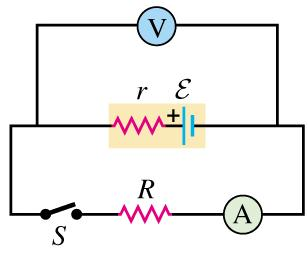a) Find the emf.

b) Find the internal resistance of the battery

c) Find the circuit resistance R.

Concepts and reason

The concept related to solve this problem is the internal resistance and terminal voltage. The concept of internal resistance is useful in analyzing many types of the electrical circuits and this concept applies to all kinds of electrical sources. First calculate the Emf of the battery. After that, calculate the internal resistance of the battery. Finally, calculate the resistance in the circuit.

Fundamentals

The resistance within a battery or other voltage source is internal resistance. Internal resistance causes a drop in the source voltage when there is a current. Electromotive force is the voltage developed by any source electrical energy.

The net resistance of the circuit when the resistor R and the internal resistance r are connected in series is as follows:

${R_{{\rm{net}}}} = R + r$

Here, R is the resistance of the resistor and r is the internal resistance.

According to the ohm’s law, the potential difference is as follows:

$V = IR$

Here, V is the potential and I is the current.

The expression for the terminal voltage is as follows:

${V_{\rm{t}}} = \varepsilon - Ir$

Here, $\varepsilon$ is the emf.

The current in the circuit is as follows:

$I = \frac{\varepsilon }{{R + r}}$

(a)

The current passing through the circuit when switch is in open position is equal to zero.

$I = 0$

Substitute $0$for$I$in the equation$V = \varepsilon - Ir$and solve for $\varepsilon$.

$\begin{array}{c}\\V = \varepsilon - \left( 0 \right)r\\\\\varepsilon = V\\\end{array}$

Substitute 3.08 V for$V$.

$\begin{array}{c}\\\varepsilon = V\\\\ = 3.08\;{\rm{V}}\\\end{array}$

The open circuit voltmeter reading is equivalent to the emf of the battery. Hence, emf of the battery is equal to 3.08 V.

(b)

The expression for the terminal voltage is as follows:

${V_{\rm{t}}} = \varepsilon - Ir$

Rewrite the equation to solve for r.

$r = \frac{{\varepsilon - {V_{\rm{t}}}}}{I}$

Substitute 3.08 V for $\varepsilon$, 2.98 V for ${V_{\rm{t}}}$, 1.64 A for I.

$\begin{array}{c}\\r = \frac{{3.08{\rm{ V}} - 2.98{\rm{ V}}}}{{1.64{\rm{ A}}}}\\\\ = \frac{{0.1{\rm{ V}}}}{{1.64{\rm{ A}}}}\\\\ = 0.061{\rm{ }}\Omega \\\end{array}$

(c)

The current in the circuit is equal to,

$I = \frac{\varepsilon }{{R + r}}$

Rewrite this equation and solve for the circuit resistance.

$R = \frac{\varepsilon }{I} - r$

Substitute 3.08 V for$\varepsilon$, 1.64 A for I, and$0.061{\rm{ }}\Omega$ for r.

$\begin{array}{c}\\R = \frac{{3.08{\rm{ V}}}}{{1.64{\rm{ A}}}} - 0.061{\rm{ }}\Omega \\\\ = 1.817{\rm{ }}\Omega \\\end{array}$

Ans: Part a

The emf of the battery is 3.08 V.

Part b

The internal resistance of the battery is equal to $0.061{\rm{ }}\Omega$.

Part c

The circuit resistance is equal to $1.817{\rm{ }}\Omega$.

#### Earn Coin

Coins can be redeemed for fabulous gifts.

Similar Homework Help Questions
• ### When switch S in the figure (Figure 1)is open, the voltmeter V of the battery reads...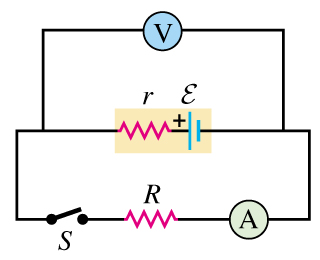When switch S in the figure (Figure 1)is open, the voltmeter V of the battery reads 3.13 V . When the switch is closed, the voltmeter reading drops to 2.99 V , and the ammeter A reads 1.70 A . Assume that the two meters are ideal, so they don't affect the circuit. 1) Find the emf. 2) Find the internal resistance of the battery. 3) Find the circuit resistance R. www d. 3

• ### *** Problem 3 When switch S in Figure 1 is open, the voltmeter V reads 3.08...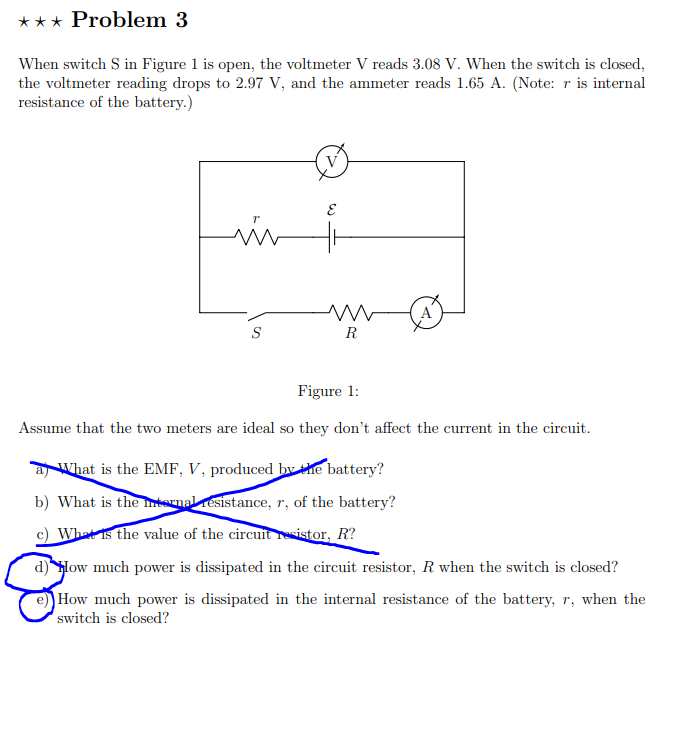*** Problem 3 When switch S in Figure 1 is open, the voltmeter V reads 3.08 V. When the switch is closed, the voltmeter reading drops to 2.97 V, and the ammeter reads 1.65 A. (Note: r is internal resistance of the battery.) fr Figure 1: Assume that the two meters are ideal so they don't affect the current in the circuit. a What is the EMF, V, produced by the battery? b) What is the liternal cesistance, r, of...

• ### When switch S in the figure is open, the voltmeter V of the battery reads 3.09...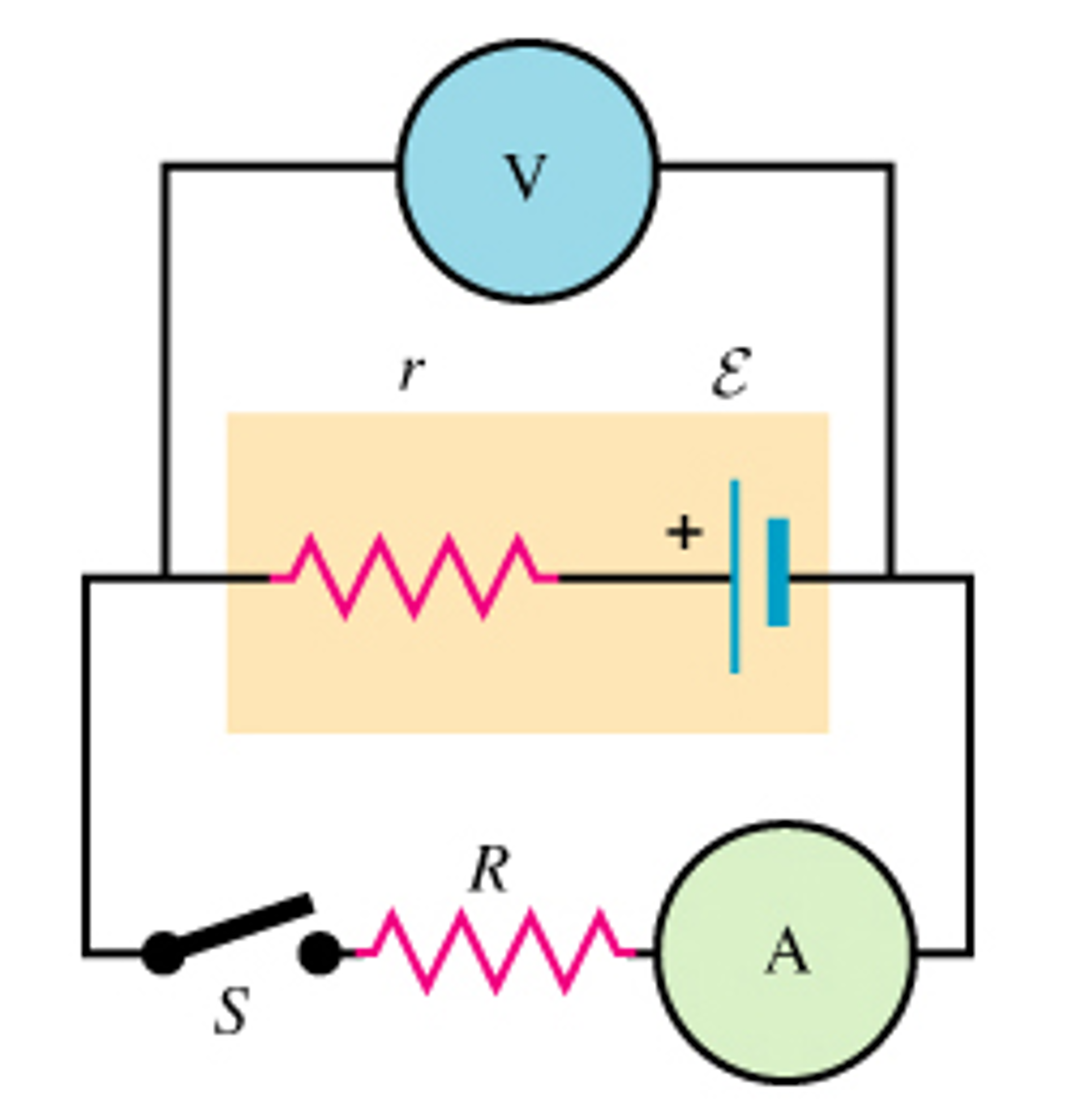When switch S in the figure is open, the voltmeter V of the battery reads 3.09 V . When the switch is closed, the voltmeter reading drops to 2.96 V , and the ammeter A reads 1.66 A . Assume that the two meters are ideal, so they do not affect the circuit. (Figure 1) - From that we find that emf E = 3.09 V Find the internal resistance r of the battery.

• ### When switch S in the figure is open, the voltmeter V of the battery reads 3.13V

When switch S in the figure is open, the voltmeter V of the battery reads 3.13V . When the switch is closed, the voltmeter reading drops to 2.90V , and the ammeter A reads 1.70A. Assume that the two meters are ideal, so they do not affect the circuit. emf= V sorry I left out the V and the A earlier.

• ### When switch S is open, the voltmeter V of the battery reads 3.13

When switch S is open, the voltmeter V of the battery reads 3.13 . When the switch is closed, the voltmeter reading drops to 2.90 , and the ammeter A reads 1.70 . Assume that the two meters are ideal, so they do not affect the circuit. emf= V

• ### Exercise 25.37

When switch S in the figure is open, the voltmeter V of the battery reads 3.11 {rm V}. When the switch is closed, the voltmeter reading drops to 2.96 {rm V}, and theammeter A reads 1.64 {rm A}. Assume that the two meters are ideal, so they don't affect the circuit.Part AFind the emf.Part BFind the internal resistance of the battery.r =Part CFind the circuit resistance R.R =

• ### emf

When switch S in the figure is open, the voltmeter V of the battery reads 3.05V . When the switch is closed, the voltmeter reading drops to 2.93V , and the ammeter Areads 1.65A . Assume that the two meters are ideal, so they do not affect the circuit. Find the emf .

• ### Batteries are not perfect. They can't deliver infinite current. As the current load on a battery...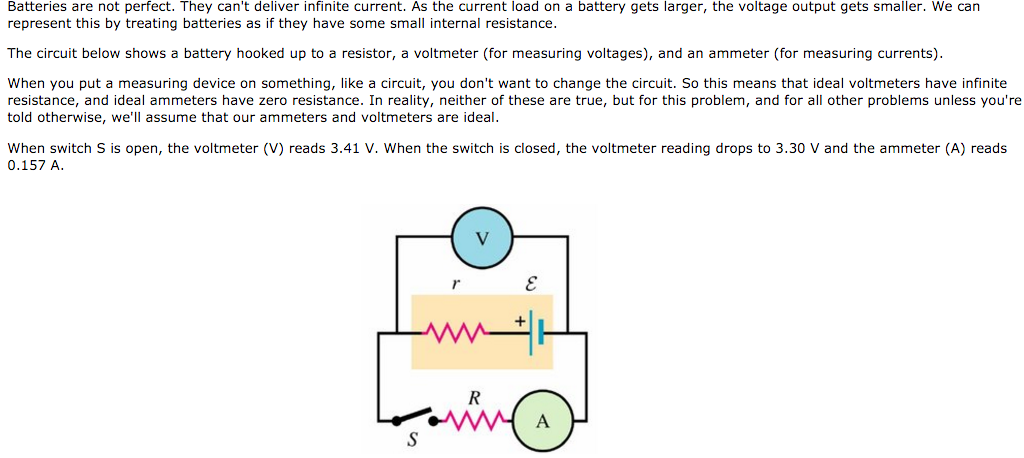Batteries are not perfect. They can't deliver infinite current. As the current load on a battery gets larger, the voltage output gets smaller. We can represent this by treating batteries as if they have some small internal resistance. The circuit below shows a battery hooked up to a resistor, a voltmeter (for measuring voltages), and an ammeter (for measuring currents). When you put a measuring device on something, like a circuit, you don't want to change the circuit. So this...

• ### In the circuit shown in the figure (Figure 1) the batteries have negligible internal resistance and...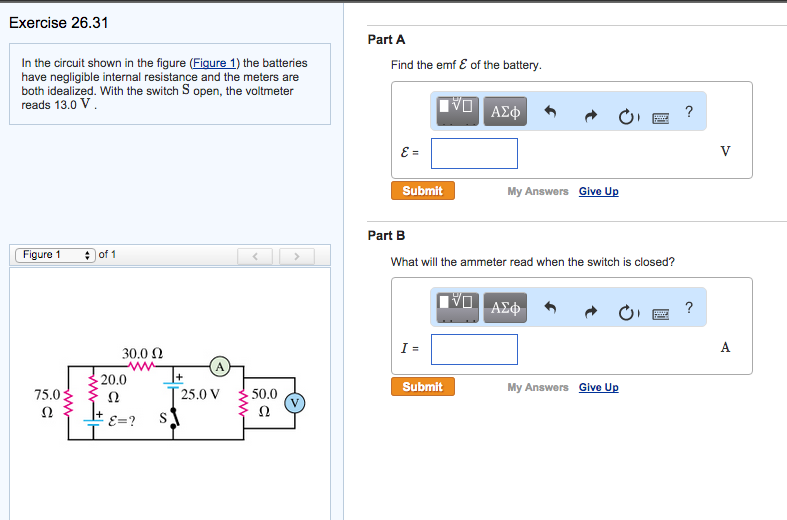In the circuit shown in the figure (Figure 1) the batteries have negligible internal resistance and the meters are both idealized. With the switch S open, the voltmeter reads 13.0 V. Find the emf epsilon of the battery. What will the ammeter read when the switch is closed?

• ### Print ViewMeasuring the EMF and Internal Resistance of a Battery

When switch S in the figure is open, thevoltmeter V of the battery reads 3.05 . When the switch is closed, the voltmeter readingdrops to 2.94, and the ammeter A reads 1.62 . Assume that the two meters are ideal, sothey donot affect the circuit.Find the internal resistance of the battery.I am not quite certain what equation I am goingto use.